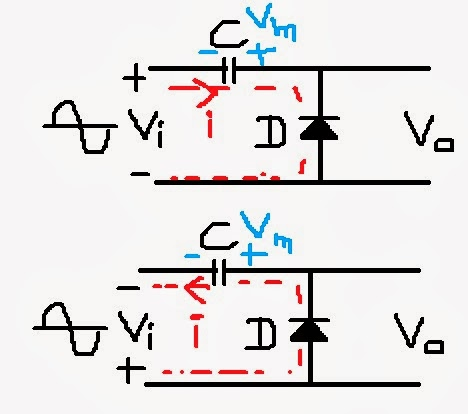### Voltage Multiplier Theory Circuit Diagram Designing (ppt and pdf) - Voltage Doubler

Searching the books for your studies can be so easy
You can search in the search bar for your book .........................................................................

Voltage Multiplier is a circuit which multiplies the voltage applied to its input. If you have come to this article after searching for the information about the voltage multiplier or voltage doublers circuit than you have visited the right page. Instead of further irritating you I must start telling you the story about our circuit of voltage doubler. Let me first give you the brief introduction of voltage multiplier circuit.

Let's say you have input voltage whose maximum value is 12V and you want to have voltage of 24V, what will you do to achieve this? You will try to multiply the 12V voltage with 2 to get 24V at the output. Hence when a circuit multiplies the input voltage by 2, that circuit is known as voltage doubler circuit.
Similarly if you want to get the 3 times of input voltage, than the circuit is known as voltage tripler circuit. The above said introduction is little complex for you than consider it simply that voltage doubler circuit doubles the input voltage.

How to achieve the voltage doubling? What is the circuit of voltage doubler looks like? How to design the voltage doubler circuit?

The answers to all these questions are provided below in the paragraphs. You just need to keep calm and keep on reading the remaining article and I promise by the end of this article you will have your most of the doubts clear about the voltage doubler circuit.

Voltage doubler circuit designing and theory is given below:
The basic components that are required for the designing of voltage doubler circuits are diode, capacitors and resistor. Diode and capacitor are the basic components which you are already familiar with. Diode acts as a switch in voltage doubler circuit for the positive and negative half cycles applied at its input. Capacitor is a charge storing device. It charges to its maximum value and after the complete charging it stops the further charging.Voltage multiplier or voltage doubler circuit diagram

Voltage multiplier Circuit diagram shows the diagram of voltage multiplier circuit. During the negative half cycle capacitor gets charged to maximum input voltage V­m. The polarity of charged voltage across the capacitor is shown in figure. During the positive half cycle, the diode is reverse biased. When the diode is reversed biased, it acts as an off switch. The voltage charged on capacitor ads with input voltage and to get the double of input voltage at the output.

In the above paragraph the theory of voltage multiplier circuit working is described. The above paragraph may be little boring to understand the concept of working of the voltage multiplier circuit. The main point to remember is that capacitor charges to the maximum voltage and this capacitor voltage ads with the input voltage to provide the double voltage at the input.

Voltage Tripler circuit is also similar to the voltage doubler circuit, but here the components increase as the multiplication factor. For voltage tripler two diodes and two capacitors are required to be connected in the circuit.

Students often search for the pdf copy of the voltage doubler topic. Most popular search terms include it like this one "voltage doubler pdf". People who are searching for the pdf copy of the voltage doubler circuit can use the copy paste method and later on save their own document to save it as a pdf copy.

For the voltage multiplier ppt, the main information points and circuit diagram can be taken from the copyright free website. From my post you can copy the image for the circuit diagram of the voltage multiplier circuit. You can even take out some important points from the discussion that we are doing in the post. The important meaningful points can be compiled with the circuit diagram to for the voltage multiplier ppt.